## Ideal

A subset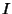of elements in a Ring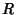which forms an additive Group and has the property that, wheneverbelongs toandbelongs to, then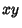and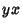belong to. For example, the set of Even Integers is an ideal in the Ring of Integers. Given an ideal, it is possible to define a Factor Ring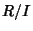.

An ideal may be viewed as a lattice and specified as the finite list of algebraic integers that form a basis for the lattice. Any two bases for the same lattice are equivalent. Ideals have multiplication, and this is basically the Kronecker product of the two bases.

For any ideal, there is an ideal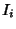such that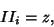where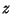is a Principal Ideal, (i.e., an ideal of rank 1). Moreover there is a finite list of idealssuch that this equation may be satisfied for every. The size of this list is known as the Class Number. In effect, the above relation imposes an Equivalence Relation on ideals, and the number of ideals modulo this relation is the class number. When the Class Number is 1, the corresponding number Ring has unique factorization and, in a sense, the class number is a measure of the failure of unique factorization in the original number ring.

Dedekind(1871) showed that every Nonzero ideal in the domain of Integers of a Field is a unique product of Prime Ideals.

See also Class Number, Divisor Theory, Ideal Number, Maximal Ideal, Prime Ideal, Principal Ideal

References

Malgrange, B. Ideals of Differentiable Functions. London: Oxford University Press, 1966.